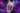View all posts

### Posts tagged with spaceBlog - development

#### Javascript: Introduction to Time Complexity

According to Wikipedia, the computational complexity, or simply the complexity of an algorithm is the number of resources required for running it. The amount of required resources varies based on the input size, so the complexity is generally expressed as a function of (n), where (n) is the size of the input.

According to Wikipedia, the computational complexity, or simply the complexity of an algorithm is the number of resources required for running it. The...